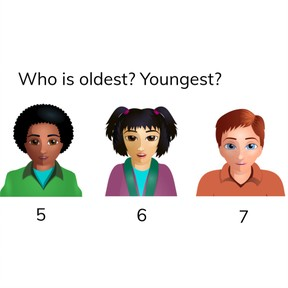Comparing and ordering numbers to 10

# Comparing and ordering numbers to 10

Comparing and ordering numbers to 10

No account needed.8,000 schools use Gynzy92,000 teachers use Gynzy1,600,000 students use Gynzy

## General

Students learn to compare the numbers to 10 and are able to put them in the correct order. That means they also know which is more and less.

K.CC.C.6
K.CC.C.7

## Introduction

Ask a few students to come to the front of the class. Divide them into groups of differing sizes smaller than 10. Ask the class what the correct order of groups is from least to most. Then ask a few students to come up to the front and hold four pencils. Ask the students if there are enough pencils for the students at the front of the class.

## Instruction

Show the number line on the interactive whiteboard and show students that one number is more than another. Explain that you can set grouped objects into numerical order- from least to most. Do this using the number line on the interactive whiteboard. Tell students that you first search for the smallest number in the grouping. The next step is to find the next smallest number. You do so by finding which number comes after the first number on the number line. The last number is the biggest number, which also comes last on the number line. Compare a few numbers as a class and set them in the right order.

To check that students can compare and order numbers to 10 you can ask the following questions:
- How do you set numbers in the right order?
- When is one number bigger than another number?
- How do you know which number is the least or the smallest?
- How do you know which number is the most or the biggest?
- Which tool can you use to help set numbers in their correct order?

## Quiz

Students first practice with numbers where they should recognize the least or most. Next they practice setting three numbers in order from least to most.

## Closing

Discuss with students that it is important to be able to compare numbers and set them in the right order so you know who is older. Check that students know how to set numbers in the correct order and from least to most. Ask if students know which number is the least and the most.

## Teaching tips

Students who have difficulty comparing and ordering numbers can practice using the number line. By pointing at the numbers on the number line, they see that the one number comes before or after another number, showing it is more or less.

## Instruction materials

pencils and number cards from 1 to 10.

### The online teaching platform for interactive whiteboards and displays in schools

• Save time building lessons

• Manage the classroom more efficiently

• Increase student engagement